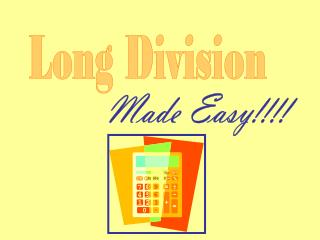DownloadDownload PresentationLong Division

# Long Division

Télécharger la présentation## Long Division

- - - - - - - - - - - - - - - - - - - - - - - - - - - E N D - - - - - - - - - - - - - - - - - - - - - - - - - - -
##### Presentation Transcript

2. ) 5 36,987 What is this problem asking us? If we divided 36,987 into 5groups of equal size, how many would be in each group?

3. ) 5 36,987 Real Life Example Your grandmother just won the lottery for \$36,987but wants to split that amount equally with her 5 grandchildren.

4. ) 5 36,987 divisor How do we solve it? dividend • 5 is our divisor • Look at the first number in the dividend • Ask how many 5s are in 3 • 5 cannot go into 3, so we ask how many 5s are in 36

5. ) 5 36,987 • How many 5s are in 36? • Recall your multiplication facts to find the one that will give an answer near 36. • 5 x 7 = 35

6. ) 5 36,987 • 5 x 7 = 35 7 • Place the 7 above the division bar • Now multiply the 7 with the divisor (5) and place the answer underneath the 36 • Subtract 35 from 36 • Bring down the next digit in the dividend 35 1 9 • Now ask how many 5s are in 19

7. ) 5 36,987 • 5 x 3 = 15 7 3 • Place the 3 above the division bar • Now multiply the 3 with the divisor (5) and place the answer underneath the 19 • Subtract 15 from 19 • Bring down the next digit in the dividend 35 9 1 15 4 8 • Now we ask how many 5s are in 48

8. ) 5 36,987 • 5 x 9 = 45 7 3 9 • Place the 9 above the division bar • Now multiply the 9 with the divisor (5) and place the answer underneath the 48 • Subtract 45 from 48 • Bring down the next digit in the dividend 35 1 9 15 48 45 • Now we ask how many 5s are in 37 3 7

9. ) 5 36,987 remainder 7 3 9 7 • Place the 7 above the division bar • Subtract 35 from 37 • There are no more numbers to bring down • The remainder is 2, which means 2 are left over • The quotient or answer to our problem is 7,397 with a remainder of 2 35 1 9 15 48 45 3 7 5 x 7 = 35 35 2

10. ) 5 36,987 Our Real Life Example 7,397 So, each of the 5 grandchildren would receive \$7,397.00 and there would be \$2.00 left over.